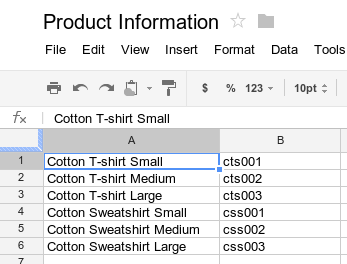## 开始使用

### 读取数据``````function logProductInfo() {
var data = sheet.getDataRange().getValues();
for (var i = 0; i < data.length; i++) {
Logger.log('Product name: ' + data[i]);
Logger.log('Product number: ' + data[i]);
}
}
``````

### 写入数据

``````function addProduct() {
sheet.appendRow(['Cotton Sweatshirt XL', 'css004']);
}
``````

## 格式设置

`Range` 类具有 `setBackground(color)` 等方法，可用于访问和修改单元格或单元格范围的格式。以下示例展示了如何设置范围的字体样式：

``````function formatMySpreadsheet() {
// Set the font style of the cells in the range of B2:C2 to be italic.
var sheet = ss.getSheets();
var cell = sheet.getRange('B2:C2');
cell.setFontStyle('italic');
}
``````

## 数据验证

``````function validateMySpreadsheet() {
// Set a rule for the cell B4 to be a number between 1 and 100.
.requireNumberBetween(1, 100)
.setAllowInvalid(false)
.setHelpText('Number must be between 1 and 100.')
.build();
cell.setDataValidation(rule);
}
``````

## 图表

``````function newChart() {
// Generate a chart representing the data in the range of A1:B15.
var sheet = ss.getSheets();

var chart = sheet.newChart()
.setChartType(Charts.ChartType.BAR)
.setPosition(5, 5, 0, 0)
.build();

sheet.insertChart(chart);
}
``````